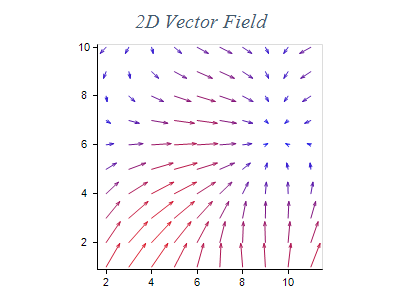﻿ Vector Series
Nevron Open Vision Documentation
Chart / Charts / Types / Cartesian / Series / Vector Series
In This Topic
Vector Series
In This Topic

Vector charts display vectors defined by two points (begin and end) which are represented as an arrow. An arrowhead is placed at the end point to indicate the vector direction. The following image shows a vector chart:Creating a Vector Series

Stock series are represented by the NVectorSeries type. An instance of this type must be added to the series collection of a Cartesian chart:

C#
Copy Code

NVectorSeries vector = new NVectorSeries();

Passing Data

Range series accept data points of type NVectorDataPoint. The following code snippet shows how to add data to a vector series:

C#
Copy Code

vector.UseXValues = true;

NVectorDataPoint vectorDataPoint = new NVectorDataPoint();

vectorDataPoint.X = 0;

vectorDataPoint.Y = 0;

vectorDataPoint.X2 = 1;

vectorDataPoint.Y2 = 1;

The width and height of the vector arrowhead are relative to the vector length, so that shorter vectors have smaller arrowheads and longer vectors have larger arrowheads. The sizes are controlled through the ArrowheadSizeFactor properties. The MinArrowHeadSize and MaxArrowHeadSize properties can be used to set minimum and maximum sizes of the arrowhead.

Formatting Commands

The vector series supports the following formatting commands in addition to the standard (per data point) formatting commands:

<y1> - displays the vector y1 value. Has the same effect as the <value> command.
<x1> - displays the vector x1 values. Has the same effect as the <xvalue> command.

<x2> - displays the vector x2 value.
<y2> - displays the vector y2 value.